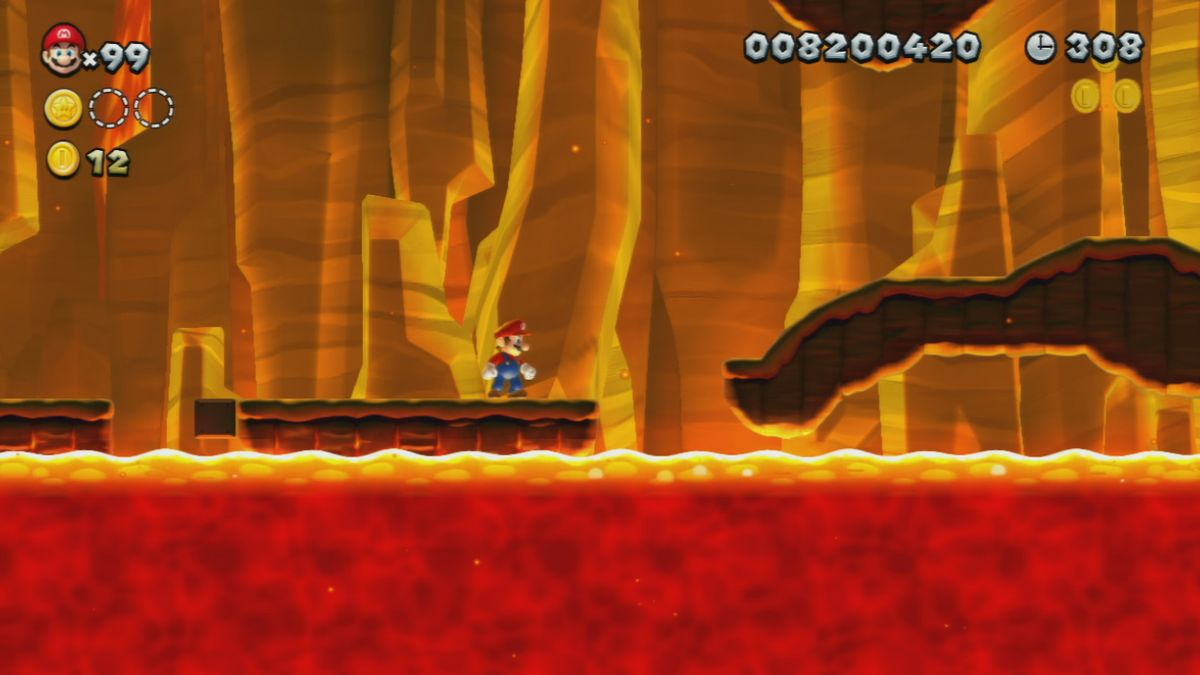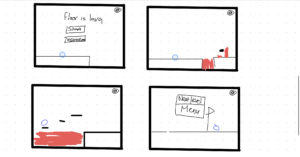# Aisha – Floor is Lava Midterm project

For my midterm project, I decided I wanted to make a floor is lava type of game where the player has to move from left to right without touching lava. There will be platforms in the game that can help the user as they can jump on it thus avoiding the lava. Once the user finishes a level they will have an option to go to the next level or back to the menu. At the beginning of the game, there will be the main menu where the start and instructions buttons are displayed. Furthermore, I might add background music or a sound effect when the user jumps (or both) to the game but there will be an option for the user to turn off the sound.

The user will move at a constant velocity from left to right. The user can press the up arrow button to allow the shape to jump. The background will be moving to the left to allow the image to scroll.

Inspirations:

I was inspired by games such as geometry dash and super Mario.

Geometry dash:

Super Mario:Rough Sketch of the game:This is all I have done so far:

```let gravity = 0.5;

class Player {
constructor() {
this.x = 100;
this.y = 320;
this.d = 30;
this.v = 0.05;
//gravity
this.xg = 0;
this.yg = 0;
}

draw() {
fill(15, 192, 252);
stroke(0, 255, 255);
ellipse(this.x, this.y, this.d);
}

update() {
this.y += this.yg;

if (this.y + this.d + this.yg <= height) {
this.yg += gravity;
} else {
this.yg = 0;
}

// if (this.x < 400){
//   this.x += this.xg;
//   this.xg += this.v;
// }
}
}

class Platform {
constructor(x,y) {
this.x = x;
this.y = y;
this.w = 100;
this.h = 20;
}

draw() {
fill(0);
rect(this.x, this.y, this.w, this.h);
}
}

let player;
let platforms = [];

function setup() {
createCanvas(800, 400);
player = new Player();
for (let i = 0; i < 100; i++) {
platforms[i] = new Platform(i + 500, 300);
}
}

function draw() {
background(230, 143, 172);
player.update();
player.draw();
for (let i = 0; i < 10; i++) {
platforms[i].draw();
}

if (player.x < 400) {
player.x += player.xg;
player.xg += player.v;
for (let i = 0; i < 10; i++) {
platforms[i].x -= 5;
}
}

// platform condition

for (let i = 0; i < 10; i++) {
if (
player.y + player.d <= platforms[i].y &&
player.y + player.d + player.yg >= platforms[i].y &&
player.x + player.d >= platforms[i].x &&
player.x <= platforms[i].x + platforms[i].w
) {
player.yg = 0;
}
}
}

function keyPressed() {
if (keyCode === UP_ARROW) {
player.yg -= 10;
}
}```

There is obviously still much more I need to do however this was a quick test run I did to make sure I know what’s going on.